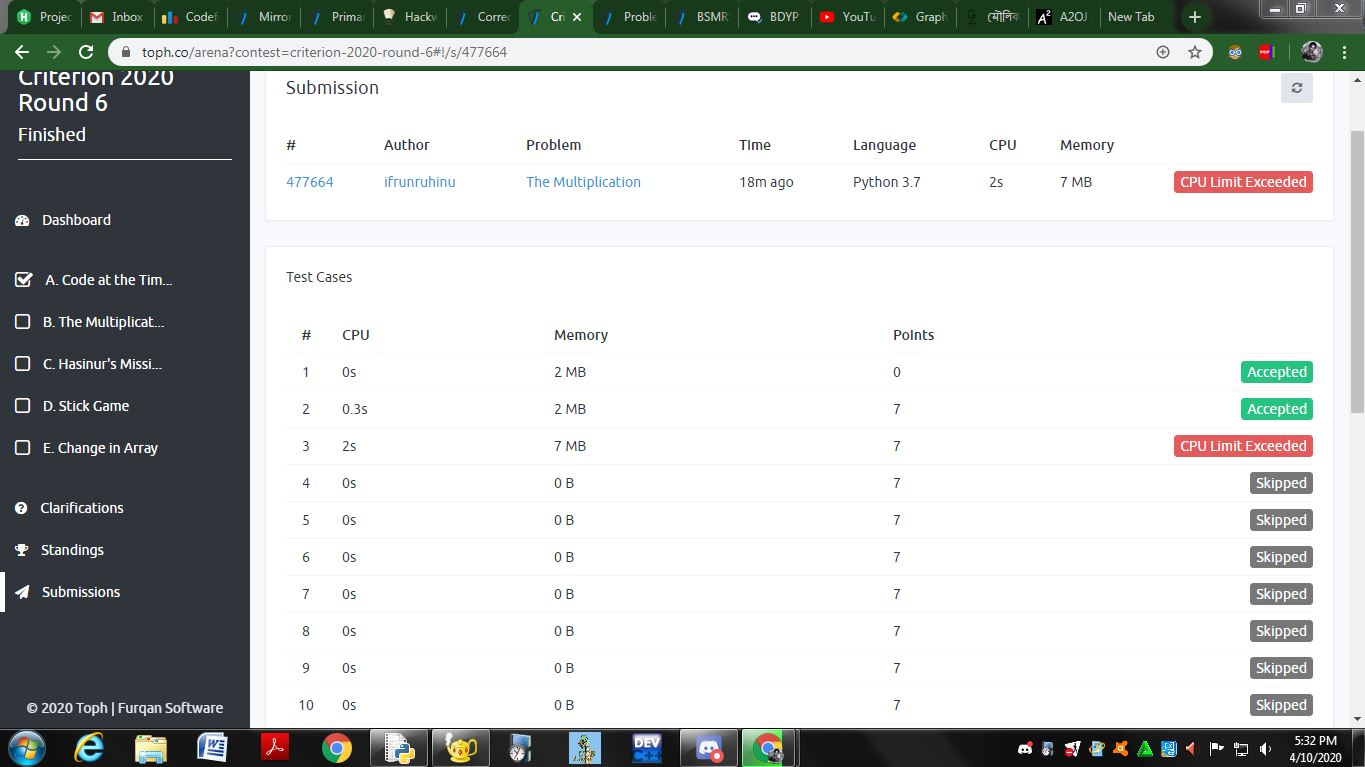# The Multiplication

Nafis is working on a mathematical project. But he is stuck on a step of it. In this step, numerous multiplications need to be performed. He needs a lot of time to do this. But as there’s a shortage of time, there’s no scope of spending a lot of time on this one task. So, he is asking you to do the task in a short amount of time.

This is a companion discussion topic for the original entry at https://toph.co/p/the-multiplication

Why my code got TLE?
Here is my code for this problem:

``````def multi(myList) :
result = 1
for x in myList:
result *= x
return result
t = int(input())
for i in range(t):
k,n=map(int,input().split())
x = 1
s=[]
while True:
s.append(1+x*k)
if x*k>n:
break
x += 1
s.pop()
print('Case '+str(i+1)+': '+str(multi(s)%(10**9+7)))
``````

Result:I optimize my code. But it got TLE.
Can anyone describe me why?
@hjr265

In each of the test cases, you are running a loop to calculate the answer. This is not well optimized. It is natural that you’re getting CLE verdict. See the editorial.
By the way, “227” in the editorial is actually “227”. I think that’s formating or typing mistake.

1 Like

Thanks, @ShadowMe! The editorial has been updated.